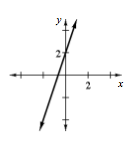### Home > CCA2 > Chapter 2 > Lesson 2.2.1 > Problem2-89

2-89.

Consider a line with a slope of $3$ and a $y$-intercept at $(0,2)$

1. Sketch the graph of this line.2. Write the equation of the line.

Try using a slope-intercept equation.
$y=mx+b$

Use the slope and $y$-intercept to make the equation.
$y=3x+2$

3. Find the initial term and the next three terms of the sequence $t(n)=3n−1$. Plot the terms on a new set of axes next to your graph from part (a) above.

Substitute $1,2,3$, and $4$ for $n$ to find the terms.
$t(1)=3-1,\ t(2)=6-1,\ t(3)=9-1,\ t(4)=12-1$

Graph the results.

$t(1)=2,\ t(2)=5,\ t(3)=8,\ t(4)=11$

4. Explain the similarities and differences between the graphs and equations in parts (a) through (c). Are both continuous?

Notice that both graphs have the same slope.

The graphs are parallel, but they have different intercepts. The first is continuous and the second is discrete.

Use the eTool below to graph the line.
Click the link at right for the full version of the eTool: CCA2 2-89 HW eTool